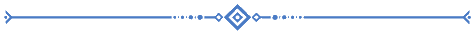# Printable Simple Subtraction Worksheets - Style 5

At Template Trove, we provide simple subtraction worksheets for students starting to learn subtraction. Our math worksheets are printable and include answer sheets for you or your student to check the correct answers.

The simple subtraction worksheet style 4 includes 54 one-digit problems for your child or pupil to solve. The simple subtraction worksheet style 5 includes 54 one-digit problems for your child or pupil to solve.

Prints on standard 8 1/2" x 11" paper on most inkjet and laser printers.

Printable Simple Subtraction Worksheet #5

Printable Simple Subtraction Worksheet #5-2

Printable Simple Subtraction Worksheet #4-3

Printable Simple Subtraction Worksheet #5-4